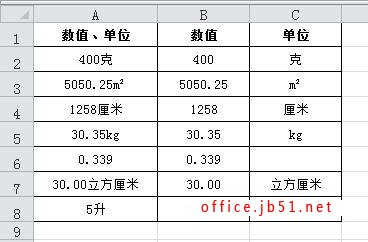Excel中如何将同一单元格内的数值和单位分离以便数值参与运算

Excel表格在设计的过程中一般会将数值及其单位放在不同的单元格内，这样做的目的是为了数值可以更方便的参与运算。不过还是有些小白们会忘记这样做，总会把单位和数值放在同一单元格内，例如“50克”、“23.55平方厘米”等等。如此一来就不能对其进行运算了，遇到这种情况该怎么解决呢？如何将其分离并放到不同的单元格中，经搜索研究发现，我们可以使用公式来实现，我们可以看到下图的A列就是这样情况。有类似需求的朋友可以参考本文。=LEFT(A2,SUMPRODUCT(--ISNUMBER(--LEFT(A2,ROW(INDIRECT("1:"&LEN(A2)))))))

=LEFT(A2,COUNT(--LEFT(A2,ROW(INDIRECT("1:"&LEN(A2))))))

=SUBSTITUTE(A2,B2,"")